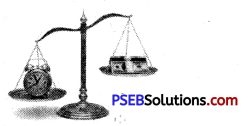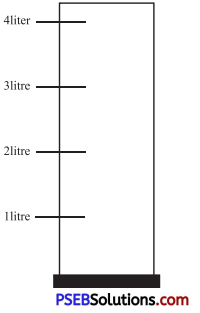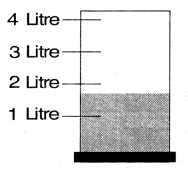# PSEB 4th Class Maths Solutions Chapter 5 Measurement Revision Exercise

Punjab State Board PSEB 4th Class Maths Book Solutions Chapter 5 Measurement Revision Exercise Questions and Answers.

## PSEB Solutions for Class 4 Maths Chapter 5 Measurement Revision Exercise

Question 1.
The length of pencil is 19 ………
(centimetre, kilogram, metre)
Solution:
Centimeter

Question 2.
Weight of a brick is 3 ……..
(litre, kilogram, metre)
KilogramQuestion 3.
There is 2 …….. water in the jug.
(litre, kilogram, metre)
Solution:
Litre

Question 4.
Draw a picture on weighing scale showing less and more weight.Solution:Question 5.
Colour the given container having capacity upto 2 litreSolution: